# Train expres

At 8.00 strarted from Bratislava to Poprad express Tatry. Cities are distant 340 kilometers. At the same time started from Poprad express Danube to Bratislava. Express Tatry's average speed is 80 km/h, Danube 90 km/h. At what distance from Poprad trains will meet?

Result

x =  180 km

#### Solution:

80t + 90t = 340
x = 90t

170t = 340
90t-x = 0

t = 2
x = 180

Calculated by our linear equations calculator.

Leave us a comment of example and its solution (i.e. if it is still somewhat unclear...):

Showing 0 comments:Be the first to comment!#### To solve this example are needed these knowledge from mathematics:

Do you have a linear equation or system of equations and looking for its solution? Or do you have quadratic equation? Do you want to convert length units?

## Next similar examples:

1. StormSo far, a storm has traveled 35 miles in 1/2 hour in direction straight to observer. If it is currently 5:00 p. m. and the storm is 105 miles away from you, at what time will the storm reach you? Explain how you solved the problem.
2. Truck and car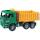At 6h45min the truck started at a speed of 36 km/h. At 7h30m behind it a passenger car. Find the average speed of passenger car if it will catch truck 72 km away from the factory.
3. Call of Duty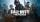Game Call of Duty in CTF (Capture the Flag) opposes the British and the Germans, whose bases are 15 km apart. A German soldier stole the British flag and hid it with it in a secret place between the bases. If the British soldiers set out at a speed of 14.
4. Steamer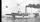At 6 hours 40 minutes steamer sailed from the port at speed 12 km/h. At exactly 10 hours started sail motorboat at speed 42 km/h. When motorboat will catch steamer?
5. Round-tripA woman works at a law firm in city A which is about 50 miles from city B. She must go to the law library in city B to get a document. Find how long it takes her to drive​ round-trip if she averages 40 mph.
6. Cars 6At 9:00 am two cars started from the same town and traveled at a rate of 35 miles per hour and the other car traveled at a rate of 40 miles per hour. After how many hours will the cars be 30 miles apart?
7. Father and sonFather is 48 years old, son 23. When will be the father twice older than a son?
8. EquationSolve the equation: 1/2-2/8 = 1/10; Write the result as a decimal number.
9. Bob traveled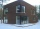Bob traveled 20 meters to Dave's house. He arrived in 3 minutes. What was his average speed for the trip.
10. Unknown numberIdentify unknown number which 1/5 is 40 greater than one tenth of that number.
11. The ship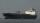The ship went upstream speed of 20 km/h in relation to water. River flows at speed 10 km/h. After half an hour, he stopped and returned downstream to the starting point. How long it took way back when even if downstream speed of ship is 20 km/h in relation
12. WayIf the current way to school was 2 km shorter then if Milan swipe one half he will have already one quarter of the way to school behind. How long is Milan's way to school?
13. Fifth of the numberThe fifth of the number is by 24 less than that number. What is the number?
14. Six yearsIn six years Jan will be twice as old as he was six years ago. How old is he?
15. Simple equationSolve for x: 3(x + 2) = x - 18
16. CandyPeter had a sachet of candy. He wanted to share with his friends. If he gave them 30 candies, he would have 62 candies. If he gave them 40 candies, he would miss 8 candies. How many friends did Peter have?
17. Pedestrian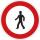Pedestrian came started at 8h in the morning with speed 4.4 km/h. At half-past eleven cyclist started at 26 km/h same way. How many minutes take cyclist to catch up pedestrian?